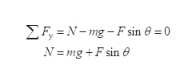# A dockworker pushes a 50 kg crate with a downward force of 300 Newton's, at an angle of 30 degrees with the horizontal. The coefficient of kinetic friction is 0.30.a. What is the normal force?b. WHat is the inetic friction once the crate starts moving?c. What is the acceleration of the crate?

Question
39 views

A dockworker pushes a 50 kg crate with a downward force of 300 Newton's, at an angle of 30 degrees with the horizontal. The coefficient of kinetic friction is 0.30.

a. What is the normal force?

b. WHat is the inetic friction once the crate starts moving?

c. What is the acceleration of the crate?

check_circle

Step 1

(a) write the expression for net vertical forcehelp_outlineImage TranscriptioncloseEF, = N– mg – F sin 0 = 0 N = mg +F sin e fullscreen
Step 2

Substitute the values

Step 3

(b) Write the expression for k...

### Want to see the full answer?

See Solution

#### Want to see this answer and more?

Solutions are written by subject experts who are available 24/7. Questions are typically answered within 1 hour.*

See Solution
*Response times may vary by subject and question.
Tagged in

### Physics